# Design and Implementation of a Student Archive Retrieval Method Based on Image Processing

Design and Implementation of a Student Archive Retrieval Method Based on Image Processing
College of Data Science and Software Engineering, Baoding University, Baoding 071000, China

Corresponding Author Email:
liaihua@bdu.edu.cn
Page:
487-494
|
DOI:
https://doi.org/10.18280/ria.340414
8 May 2020
|
Accepted:
2 August 2020
|
Published:
30 September 2020
| Citation

OPEN ACCESS

Abstract:

Compared with traditional manual archive organization and review, the student archive management system can manage massive student archives in a refined, regular, and scientific manner. The effectiveness and efficiency of the retrieval method directly bears on the utilization effect of student archives. Based on image processing, this paper puts forward a novel method for student archive retrieval, which greatly improves the classification, recognition, and information management of images in student archives during the retrieval. Firstly, a framework of student archive retrieval was introduced based on image processing. Next, a deep convolutional neural network (DCNN) was constructed for hash learning, and the functions of the three network modules were detailed, including image feature extraction, hash function learning, and similarity measurement. Finally, several indices were selected to evaluate the retrieval effect of student archives. The proposed method was proved effective and feasible through contrastive experiments. The research results provide a theoretical reference for the application of our method in other fields of image retrieval.

Keywords:

image processing, archive retrieval, hash learning, deep convolutional neural network (DCNN)

1. Introduction

Student archive management system is an indispensable tool of education institutions. The main functions of the system include providing consultation to various departments, and offering reference data for student enrollment and employment [1-4]. Compared with traditional manual archive organization and review, the student archive management system has the advantages of high reliability, large storage, and good confidentiality, and manages massive student archives in a refined, regular, and scientific manner. Owing to the superiority of information technology (IT), the student archive management system promotes the informatization of archives, while supporting the relevant works of the school and the society [5-9].

Retrieval is the key operation in the use of student archives. The effectiveness and efficiency of the retrieval method directly bears on the utilization effect. Traditionally, student files are generally retrieved by subject term matching, which is easy-to-use and convenient. This traditional method applies to the retrieval of archives in structured languages like numbers and words [10-14]. Tekeste and Demir  summarized the basic measures for improving the retrieval of college student archives, highlighted the importance of refining the subject terms, and proposed to configure the search database and tabs by the vertical and horizontal classification method.

Some archives can be effectively retrieved according to the preparation rules of student archives and the indexing rules of subject terms. But this retrieval method cannot achieve satisfactory effect on image archives. With the technical progress of image processing, the image processing technology for student archives has attracted great attention [3, 15]. Boualleg and Farah  classified the images in student archives through deep learning (DL), and improved the recognition of key feature points in such images in archive retrieval, through image denoising, image fusion, and salient feature extraction. Shirazi et al.  classified the images in student archives by fast fractal automatic image coding, and constructed the corresponding image analysis model, which identifies and tracks student identity with pixel sequence distributed fusion algorithm. Through image recognition and segmentation, Sawant and Topannavar  recognized and fused the features of the images of student archives, constructed a student archive image collection model, and designed an edge feature detection algorithm, realizing the classification detection and region reconstruction of such images.

Some scholars have applied fast and accurate facial image recognition to the field of archive retrieval [9, 18]. Demir and Bruzzone  divided the facial area of student images in the local domain, and then quantized and coded the facial features; the resulting visual words were prepared into a reverse index. Next, the retrieval results were repeatedly reordered according to the global facial features, making the retrieval more accurate. Based on Public Figures Face Database (Pubfig), Tang et al.  developed a facial attribute learning and retrieval framework, which measures the face matching degree by the trained attributes and the results of the similarity classifier. Paulin et al.  improved the performance of facial image retrieval by establishing a correlation model between different facial attributes.

This paper mainly improves the ability of mainstream face retrieval methods to classify the images and manage the information of student archives. Firstly, the framework of student archive retrieval was designed based on image processing. Next, a deep convolutional neural network (DCNN) model for hash learning was created to realize the retrieval of student archives, and the modules of the model, namely, image feature extraction, hash function learning, and similarity measurement, were introduced in details. After that, the evaluation indices for student archive retrieval were selected, and used to verify the proposed method through experiments.

2. Framework of Student Archive Retrieval

There are several problems with text retrieval: the limited description ability of text, the heavy workload of manual tagging, and the individual difference in understanding. To solve these problems, this paper implements DL and hash retrieval to student archive retrieval.

## 111.pngFigure 1. The framework of student archive retrieval based on image processing

## 222.png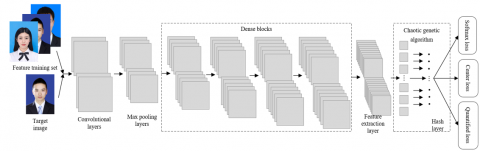Figure 2. The structure of the DCNN for hash learning

Figure 1 above illustrates the proposed framework of student archive retrieval based on image processing.

Under the proposed framework, a DCNN model is constructed and trained in the offline stage, taking the advantage of the artificial intelligence (AI) in handling massive data. Through largescale iterations on student archive images, the DCNN model can acquire the criteria that fully reflect the features of these images, that is, extracting the hash code of each image and building a hash database for local image features. At this stage, the hash function can map the features from image feature space to binary codes in Hamming space, while ensuring the similarity between the codes and the original image features. By the hash function, the high-dimensional features of each image are converted into low-dimensional binary codes (e.g. 24-bits, 32-bits, and 48-bits).

The online retrieval bears high resemblance with content-based image archive retrieval. With the help of DL, the original comparison between contents is updated into the comparison between contents and criteria. Firstly, the hash codes of the student images to be retrieved are extracted by the trained DCNN model. Then, the similarities between the image features and hash feature database are measured, and ranked in descending order, before returning the retrieval results. At this stage, the hash function converts the target images into binary hash codes. After similarity measurement, a threshold is set up, and the retrieval results are returned.

Compared with the traditional content-based retrieval method for audiovisual archives, the proposed retrieval method can characterize the essential features of images in multiple dimensions, following the criteria for the image features generated through largescale computation. The retrieval process can be divided into three parts: image feature extraction, hash function learning, and similarity measurement.

3. Hash Retrieval of Student Archive Images

The proposed retrieval method aims to preserve the semantics of student images in compact binary codes through learning and training. For effective classification of numerous student images, the binary codes must be highly distinctive, occupy a small storage, and support fast query.

Figure 2 above shows the structure of the DCNN for hash learning. Table 1 lists the parameters of the DCNN. The neural network processes each input (student image) sequentially through three functional modules:

(1) The main neural network, which contains multiple dense blocks, extracts the features from the student image.

(2) The chaotic genetic algorithm generates hash codes. This module contains convolutional layers and global average pooling layers.

(3) The combinatory loss function supervises the learning of the entire neural network.

Table 1. The parameters of the DCNN

 Layer Kernel size × Number Step length Output Convolutional layer 3×3×64 1 224×224×64 Max pooling layer 2×2 1 112×112×64 Dense block layer 3×3×256 4 56×56×128 Feature extraction layer 2×2×512 1 28×28×256 Chaotic genetic algorithm 3×3×L 1 1×1×L

3.1 Image feature extraction

The DL is a necessary technology for the extraction of the deep features from the facial images of students. Unlike traditional manual feature extraction method, the convolution operation in DCNN can intelligently discard unnecessary information, while retaining useful information. This neural network supports robust extraction of deep image features, and enhances the performance of image hash retrieval, providing a suitable tool for facial image retrieval in complex environments.

The DCNN is established by introducing pooling and convolution into traditional backpropagation neural network (BPNN). During image information processing, the pixels of the original image can be directly imported to the DCNN, without going through the tedious preprocessing steps. Our DCNN is mainly made up of input layer, convolutional layer, pooling layer, feature extraction layer, and output layer.

As the gateway of the neural network, the input layer is responsible for preprocessing the input data through cropping, flipping, color space transform, and averaging, turning the data into the format required by the other layers.

In each convolutional layer, the convolution operation is to intelligently discard unnecessary information while retaining useful information. During convolution, multiple filter windows slide on the image, and the same convolution kernel produces a set of two-dimensional (2D) images. These 2D images are merged to acquire the global information of the entire image. The convolution operation blesses the DCNN with translation-invariant local connections and weights, which effectively reduces the number of network parameters and boosts the generalization ability of the network. For the classification and recognition of archive images, the shallow convolutional layers of the DCNN mainly extract the small local features from the image, including edges, textures, and color changes.

A good representation of facial features should contain both local details and advanced semantics. Therefore, this paper optimizes each convolutional layer in the main neural network, referring to the design idea of dense blocks in DenseNet:

Step 1. Extract detailed features like edges and angles of the original image through a convolutional layer.

To extract features from the entire image, multiple filter windows are arranged to cover multiple types of basic information, because each window corresponds to only one kind of basic image features. After the multi-channel input is convoluted by multiple kernels and processed by the activation function, the map $O_{j}^{k}$ of the j-th output feature on the k-th layer can be obtained by:

$O_{j}^{k}=\operatorname{sigmoid}\left(\sum_{j \in N} I_{i}^{k-1} * w_{i j}^{k}+\varepsilon_{j}^{k}\right)$            (1)

where, $I_{i}^{k-1}$ is the map of the i-th input feature on the k-1-th layer; ${w_{i j}}^{k}$ is the kernel between the i-th input feature on the k-1-th layer and the j-th output feature on the k-th layer; $\varepsilon_{j}^{k}$ is the bias of the j-th output feature on the k-th layer; N is the number of feature maps on the k-1-th layer.

The sigmoid function is selected as the activation function:

$f(x)=\frac{1}{1+e^{-x}}$             (2)

The function can map the entire real number domain to the interval of [0, 1], facilitate derivation, and stabilize model optimization.

Step 2. Reduce the location sensitivity of the convolutional layer and the number of network parameters with a max pooling layer.

To further lower the number of network parameters, the output of the activation function is pooled to reduce the size of the feature map, the resolution of the image, and the location sensitivity of the convolutional layer. In our DCNN, the max pooling can be defined as:

$O_{j, r}^{k}=\max _{0 \leq m, n<d}\left\{I_{j \cdot d+m, r \cdot d+n}^{k}\right\}$           (3)

The average pooling can be defined as:

$O_{j, r}^{k}=\frac{1}{d^{2}} \sum_{m=0}^{d-1} \sum_{n=0}^{d-1} I_{j \cdot d+m, r \cdot d+n}^{k}$        (4)

Through a d×d pooling, the k-th input Ik can be converted into the feature map Ok outputted from the k-th layer.

Step 3. Extract multidimensional image information on the dense block layer, following the design idea of dense blocks.

To integrate multidimensional image information, the dense blocks will stitch the dimensions of the output of the previous layer and the input of the subsequence layer through feedforward method. Figure 3 explains the structure of the DenseNet.

Step 4. Obtain the feature extraction layer through the transition module, and take it as the feature output layer instead of the fully connected layer in the traditional structure.

## 3.png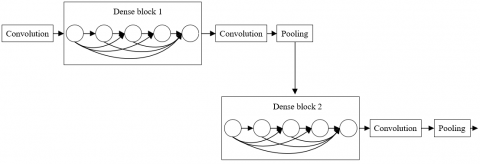Figure 3. The structure of the DenseNet

The feature extraction layer is the last but most important part of the DCNN. As shown in Figure 4, the linear combinations of the nodes on the feature extraction layer constitute the outputs of the nodes in the fully connected layer.

To reduce the number of channels and halve the height and width of the average pooling layer, the feature extraction layer combines the 1×1 convolutional layer with the average pooling layer whose step length is 2. The combination not only simplifies the model, but also makes the multidimensional feature map more robust.

## 4.pngFigure 4. The structure of the feature extraction layer

For the classification and recognition of archive images, the output layer of the DCNN is equivalent to an archive image classifier. In this layer, the network parameters are updated through the computing and backpropagation of the error between the predicted class and the actual class.

Suppose the training set contains p samples in q classes. Then, the set of samples can be expressed as {(I1, O1), (I2, O2),…,(Ip, Op)}, where Oi∈{1, 2, …, q}. Then, the probability that an input image Ii belongs to a class can be calculated by:

$P_{\alpha}\left(I^{i}\right)=\left[\begin{array}{c}h\left(O^{i}=1 \mid I^{i} ; \alpha\right) \\ h\left(O^{i}=2 \mid I^{i} ; \alpha\right) \\ \vdots \\ h\left(O^{i}=k \mid I^{i} ; \alpha\right)\end{array}\right]=\frac{1}{\sum_{j=1}^{q} e^{\alpha_{j}^{T I^{i}}}}\left[\begin{array}{c}e^{\alpha_{1}^{T} I^{i}} \\ e^{\alpha_{2}^{T} I^{i}} \\ \vdots \\ e^{\alpha_{k}^{T} I^{i}}\end{array}\right]$           (5)

where, α1, α2, …, αk are DCNN parameters. The probability that image Ii belongs to the j-th class can be calculated by:

$h\left(O^{i}=j \mid I^{i} ; \alpha\right)=\frac{e^{\alpha_{j}^{T} I^{i}}}{\sum_{j=1}^{q} e^{\alpha_{j}^{T} I^{i}}}$            (6)

The conditional probability that image Ii belongs to every other class can be calculated similarly. Then, the class corresponding to the largest probability will be taken as the predicted class of that image.

3.2 Similarity measurement

The similarity between student archive images and the standard image was measured in two approaches: (1) the measurement based on color, location, and shape; (2) the measurement with hash-based loss function.

Before computing the color similarity between images, the chromaticity coordinates (h, s, b), which represent hue, saturation, and brightness, are converted into the coordinates in Euclidean space (e1, e2, e3):

$\left\{\begin{array}{c}e_{1}=s * \cos (h) \\ e_{2}=s * \sin (h) \\ e_{3}=b\end{array}\right.$           (7)

Let $\delta_{1}$ be the variance of color feature. Then, the color image can be calculated by:

$S_{c o l}=\exp \left(\frac{\left(e_{1 i}-e_{1 j}\right)^{2}+\left(e_{2 i}-e_{2 j}\right)^{2}+\left(e_{3 i}-e_{3 j}\right)^{2}}{3 \delta_{1}^{2}}\right)$          (8)

The location feature of the image can be characterized by the centroid coordinates of the main target of the image, and normalized by:

$(\bar{a}, \bar{b})=\left(\frac{a}{W}, \frac{b}{H}\right)$         (9)

where, W is the width of the image; H is the height of the image. Let $\delta_{2}$ be the variance of location feature. Then, the location similarity can be calculated by:

$S_{p o s}=\exp \left(\frac{\left(\bar{a}_{i}-\bar{a}_{j}\right)^{2}+\left(\bar{b}_{i}-\bar{b}_{j}\right)^{2}}{2 \delta_{2}^{2}}\right)$          (10)

The shape features of the image include the size of the target as a percentage of the image area φ, and the major-minor axis ratio η of the best-fit ellipse of the image. The former reflects the shape size, while the latter indicates the image eccentricity. Let δ3 be the variance of shape features. Then, the shape similarity can be calculated by:

$S_{\text {sha}}=\exp \left(\frac{\omega_{\phi}\left(\phi_{i}-\phi_{j}\right)^{2}+\omega_{e}\left(\eta_{i}-\eta_{j}\right)^{2}}{\delta_{3}^{2}}\right)$          (11)

where, $\omega_{\varphi}$ and $\omega_{\eta}$ are the weighting coefficients of the shape size and image eccentricity, respectively.

Next, the weighted average of the color, location, and shape similarities was calculated to obtain the multi-feature similarity between an image and the standard image. Let ωcol, ωpos, and ωsha be the weighting coefficients of color, location, and shape features, respectively. Then, the multi-feature similarity between student archive image i and the standard image j can be calculated by:

$S_{1}(i, j)=\omega_{c o l} S_{c o l}+\omega_{p o s} S_{p o s}+\omega_{s h a} S_{s h a}$        (12)

Based on the user feedback on the output results, the weight of each feature in formula (11) can be adjusted adaptively, that is, enhancing the weighting coefficients of the main feature after each retrieval. Let Fi be the most similar feature between the target image and the feature database. Then, the weighting coefficient of Fi should be increased, and that of any other feature Fj should be reduced:

$\omega_{i}^{*}=\frac{\omega_{i}+\frac{1}{p}}{1+\frac{1}{p}}, w_{j}^{*}=\frac{w_{j}}{1+\frac{1}{p}}$           (13)

In this paper, the objective of hash learning is set as follows: each image Ii in student archive corresponds to a set of discrete hash codes $\beta_{i}=\{1,1\}^{L}$, where L is the length of hash code. The hash codes must be highly differentiable, facilitating the prediction of the class tag of Ii. The supervision signal of the classification can be expressed as formula (6). The loss on all p samples can be adjusted to:

$\operatorname{loss}=\sum_{i=1}^{p}-\log h\left(O^{i}=j \mid I^{i} ; \alpha\right)=\sum_{i=1}^{p}-\log \frac{e^{\alpha_{j}^{T} \beta^{i}}}{\sum_{j=1}^{q} e^{\alpha_{j}^{T} \beta^{i}}}$            (14)

To alleviate the imbalance between positive and negative samples, and reduce the time cost and space loss of training, an additional penalty called “center loss” is introduced to compress the differences between the same classes. After the addition of center loss, the loss can be expressed as:

$\operatorname{los} s^{*}=\operatorname{loss}+\gamma \operatorname{los} s_{C}=\sum_{i=1}^{p}-\log \frac{e^{\alpha_{j}^{T} \beta^{i}}}{\sum_{j=1}^{q} e^{\alpha_{j}^{T} \beta^{i}}}+\frac{\gamma}{2} \sum_{i}^{p}\left\|\beta^{i}-c_{j}\right\|_{2}^{2}$             (15)

where, γ is a hyperparameter about the relative importance of loss function; cj is the center of the j-th class. The optimization objective can be described as:

$\min \sum_{i=1}^{p}-\log \frac{e^{\alpha_{j}^{T} \beta^{i}}}{\sum_{j=1}^{q} e^{\alpha_{j}^{T} \beta^{i}}}+\frac{\gamma}{2} \sum_{i}^{p}\left\|\beta^{i}-c_{j}\right\|_{2}^{2}, s . t . \beta_{i}\in\{-1,1\}^{L}$           (16)

To solve the optimization problem with discrete constraints, the discrete vector βi is relaxed into a continuous vector. Then, the tanh function is selected to restrict all variables within the interval of [-1, 1]. After regularization, the optimization objective can be rewritten as:

$\min \sum_{i=1}^{p}-\log \frac{e^{\alpha_{j}^{T} \beta^{i}}}{\sum_{j=1}^{q} e^{\alpha_{j}^{T} \beta^{i}}}+\frac{\gamma}{2} \sum_{i}^{p}\left\|\beta^{i}-c_{j}\right\|_{2}^{2}+\lambda \sum_{i=1}^{p}\left\|\left|\beta^{i}\right|-1\right\| 1$            (17)

where, $\left|\beta_{\mathrm{i}}\right|$ is the absolute value of $\beta_{1}$; 1 is the vector whose elements are all 1; $\lambda$ is the weighting coefficient of regularization.

Next, the multidimensional feature map of the student image is mapped by the chaotic genetic algorithm. The chaotic genetic algorithm module contains L convolutional layers with 3×3 kernels, which produces L feature maps that integrate multidimensional information. Next, binary hash codes are generated on the global average pooling layer for each feature map. Finally, the L 1-bit hash codes are stitched into an L-bit hash code, i.e. the required hash code. The eigenvector of the L-bit hash code can be expressed as:

$\operatorname{sign}(\beta)=\left\{\begin{array}{l}1, \beta>0 \\ 0, \text { otherwise }\end{array}\right.$            (18)

4. Retrieval Indices and Experimental Verification

4.1 Retrieval indices

The retrieval results of the proposed student archive image retrieval method fall into four categories: similar images are retrieved, non-similar images are retrieved, no similar images are retrieved, and non-similar images are not retrieved. The number of retrieved images in the four categories is denoted as QYY, QYN, QNY, and QNN, respectively. Then, the actual retrieval results can be described as a confusion matrix (Table 2).

Table 2. The confusion matrix of the actual retrieval results

 Actual results Retrieved results Retrieved Not retrieved Similar images QYY QNY Non-similar images QYN QNN

Four indices were selected to evaluate the effectiveness of our retrieval method, namely, precision, recall, average precision, and mean average precision.

The precision refers to the proportion of similar images in the retrieved results:

$P R=\frac{Q_{Y Y}}{Q_{Y Y}+Q_{N Y}}$             (19)

The recall refers to the ratio of retrieved similar images to all similar images:

$R E C=\frac{Q_{Y Y}}{Q_{Y Y}+Q_{Y N}}$              (20)

The average precision refers to the average retrieval precision of a student archive image:

$A P\left(I^{i}\right)=\frac{1}{p^{\prime}} \sum_{j=1}^{p^{\prime}} \frac{u^{\prime}}{u}$            (21)

where, $u^{\prime}$ is the number of similar images in the u-th retrieval result; $p^{\prime}$ is the number of training images that belong to the same student archive as the target image Ii.

The mean average precision is the mean of the average precisions of all student archive images, which reflects the overall performance of our method:

$M A P=\frac{1}{p} \sum_{i=1}^{p} A P\left(I^{i}\right)$               (22)

4.2 Experiments and results analysis

To demonstrate the superiority of our method, contrastive experiments were carried out on different hash retrieval methods, using the electronic student archives of three colleges. The student archives of each college were compiled into a database. Together, the three databases contain 15,290 images on 15,677 students. From the databases, 10,000 student images were allocated to the training set, and the remaining 5,290 images to the test set. Every experiment was conducted under the DL framework Keras and the backend framework TensorFlow 1.9.0.

The contrastive methods include shallow hash algorithm, deep hash algorithm, and shallow hash + CNN, and deep hash + CNN. The latter two algorithms were created by importing the features extracted by CNN to shallow and deep hash algorithms, respectively. Based on the three databases, contrastive experiments were performed with hash codes of three different lengths: 24-bits, 48-bits, and 96-bits.

Table 3 compares the performance of different hash methods by mean average precision. It can be seen that our method far outperformed the other hash methods, indicating that the chaotic genetic algorithm can reduce the number of network parameters, and make the hash code distinctive, short, and concise. The results also manifest the reasonability of the end-to-end framework merged from hash algorithm and neural network.

Table 3. The mean average precisions of different hash algorithms

 Method Database 1 Database 2 Database 3 24-bits 48-bits 96-bits 24-bits 48-bits 96-bits 24-bits 48-bits 96-bits Shallow hash 0.0324 0.0511 0.0693 0.0225 0.0367 0.0692 0.0152 0.0244 0.0379 Deep hash 0.0470 0.0672 0.0800 0.0341 0.0556 0.0677 0.0334 0.0541 0.0758 Shallow hash + CNN 0.0583 0.0896 0.1252 0.0443 0.0755 0.0989 0.0422 0.0564 0.0799 Deep hash + CNN 0.0719 0.0874 0.1320 0.0625 0.0941 0.1255 0.0579 0.0798 0.1134 Our method 0.3119 0.3573 0.3785 0.0780 0.1021 0.1594 0.0798 0.1087 0.1354

To further compare the performance of the above hash methods, the recall curves of these methods are plotted (Figure 5), and the recall curves of image returned under the conditions of different hash methods are recorded (Figure 6), under the Hamming radius of 2. As shown in Figures 5 and 6, the hash methods based on neural network outputs achieved lower recalls than the methods based on manually extracted features, and our method had much better performance than other methods. The results confirm that our method can generate concise yet highly differentiable hash codes, and can be effectively applied in the retrieval of a massive amount of student archive images.

To find out why our method can produce desirable hash codes, the influence of different parts of our method on retrieval performance was compared. The retrieval performance of different neural network structures is contrasted in Table 4, where A is the DCNN, B is the DCNN + Dense Block, C is the DCNN + Dense Block + chaotic genetic algorithm, and D is the complete model proposed in this research. The results in Table 4 show that, compared with the DCNN, the DCNN + Dense Block effectively extracted the multidimensional features from student images; the DCNN + Dense Block + chaotic genetic algorithm lowers the redundancy of hash codes and reduces the number of parameters; the complete model boasts highly differentiable binary hash code, because the center loss compresses the differences between the same classes

## 5.png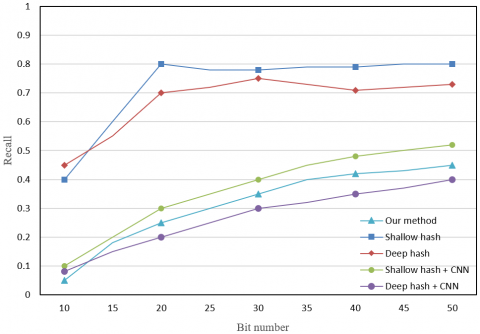Figure 5. The recall curves of different hash methods

## 6.png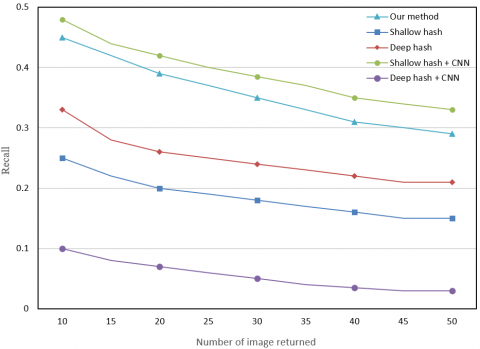Figure 6. The recall curves of image returned under the conditions of different hash methods

Table 4. The retrieval performance of different neural network structures

 Database Model Average precision Mean average precision Database 1 A 0.2974 0.3211 B 0.3163 0.3370 C 0.3343 0.3566 D 0.3519 0.3624 Database 2 A 0.0635 0.0711 B 0.0841 0.0976 C 0.0915 0.1093 D 0.1105 0.1325 Database 3 A 0.0521 0.0712 B 0.0795 0.0971 C 0.0879 0.1042 D 0.0916 0.1275

Figure 7 compares the hash features of the trained model with those of other coding methods. With the same network structure, the coding method of our model generates more differentiable and salient features of student archive images than other coding methods.

## 7.png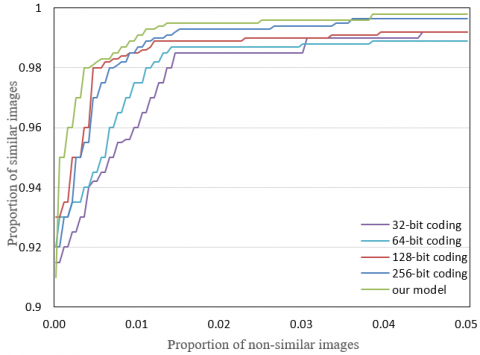Figure 7. The results on hash features

5. Conclusions

Based on mainstream face retrieval methods, this paper puts forward a novel method for student archive retrieval, which greatly improves the classification, recognition, and information management of images in student archives during the retrieval. Firstly, the framework of student archive retrieval was introduced based on image processing. Next, three important modules of the DCNN for student archive retrieval were designed, including image feature extraction, hash function learning, and similarity measurement. Finally, the evaluation metrics were defined for the retrieval effect of student archives. Experimental results show that our method far outperforms the other hash methods, and produces concise yet highly differentiable binary hash codes. The results also manifest the reasonability of the end-to-end framework merged from hash algorithm and neural network. The proposed method can be effectively applied to the retrieval of a massive amount of student archive images.

References

 Tekeste, I., Demir, B. (2018). Advanced local binary patterns for remote sensing image retrieval. In IGARSS 2018-2018 IEEE International Geoscience and Remote Sensing Symposium, pp. 6855-6858. https://doi.org/10.1109/IGARSS.2018.8518856

 Chaudhuri, B., Demir, B., Bruzzone, L., Chaudhuri, S. (2016). Region-based retrieval of remote sensing images using an unsupervised graph-theoretic approach. IEEE Geoscience and Remote Sensing Letters, 13(7): 987-991. https://doi.org/10.1109/LGRS.2016.2558289

 Demir, B., Bruzzone, L. (2015). Hashing-based scalable remote sensing image search and retrieval in large archives. IEEE Transactions on Geoscience and Remote Sensing, 54(2): 892-904. https://doi.org/10.1109/TGRS.2015.2469138

 Dai, O.E., Demir, B., Sankur, B., Bruzzone, L. (2018). A novel system for content-based retrieval of single and multi-label high-dimensional remote sensing images. IEEE Journal of Selected Topics in Applied Earth Observations and Remote Sensing, 11(7): 2473-2490. https://doi.org/10.1109/JSTARS.2018.2832985

 Boualleg, Y., Farah, M. (2018). Enhanced interactive remote sensing image retrieval with scene classification convolutional neural networks model. In IGARSS 2018-2018 IEEE International Geoscience and Remote Sensing Symposium, pp. 4748-4751. https://doi.org/10.1109/IGARSS.2018.8518388

 Roy, S., Sangineto, E., Demir, B., Sebe, N. (2018). Deep metric and hash-code learning for content-based retrieval of remote sensing images. In IGARSS 2018-2018 IEEE International Geoscience and Remote Sensing Symposium, pp. 4539-4542. https://doi.org/10.1109/IGARSS.2018.8518381

 Shen, F., Shen, C., Shi, Q., Van den Hengel, A., Tang, Z., Shen, H.T. (2015). Hashing on nonlinear manifolds. IEEE Transactions on Image Processing, 24(6): 1839-1851. https://doi.org/10.1109/TIP.2015.2405340

 Cao, Y., Steffey, S., He, J., Xiao, D., Tao, C., Chen, P., Müller, H. (2014). Medical image retrieval: a multimodal approach. Cancer Informatics, 13: CIN-S14053. https://doi.org/10.4137/CIN.S14053

 Markonis, D., Schaer, R., Müller, H. (2016). Evaluating multimodal relevance feedback techniques for medical image retrieval. Information Retrieval Journal, 19(1-2): 100-112. https://doi.org/10.1007/s10791-015-9260-4

 Zagoruyko, S., Komodakis, N. (2015). Learning to compare image patches via convolutional neural networks. In Proceedings of the IEEE Conference on Computer Vision and Pattern Recognition, pp. 4353-4361.

 Balntas, V., Johns, E., Tang, L., Mikolajczyk, K. (2016). PN-Net: Conjoined triple deep network for learning local image descriptors. arXiv preprint arXiv:1601.05030.

 Paulin, M., Douze, M., Harchaoui, Z., Mairal, J., Perronin, F., Schmid, C. (2015). Local convolutional features with unsupervised training for image retrieval. In Proceedings of the IEEE International Conference on Computer Vision, pp. 91-99.

 Zhang, S., Yang, M., Cour, T., Yu, K., Metaxas, D.N. (2014). Query specific rank fusion for image retrieval. IEEE Transactions on Pattern Analysis and Machine Intelligence, 37(4): 803-815. https://doi.org/10.1109/TPAMI.2014.2346201

 Liu, H., Wang, R., Shan, S., Chen, X. (2016). Deep supervised hashing for fast image retrieval. In Proceedings of the IEEE Conference on Computer Vision and Pattern Recognition, pp. 2064-2072.

 Chaudhuri, B., Demir, B., Chaudhuri, S., Bruzzone, L. (2017). Multilabel remote sensing image retrieval using a semisupervised graph-theoretic method. IEEE Transactions on Geoscience and Remote Sensing, 56(2): 1144-1158. https://doi.org/10.1109/TGRS.2017.2760909

 Shirazi, S.H., Khan, N., Umar, A.I., Naz, M.R., AlHaqbani, B. (2016). Content-based image retrieval using texture color shape and region. International Journal of Advanced Computer Science and Applications, 7(1): 418-426.

 Sawant, S.S., Topannavar, P.S. (2015). Introduction to probabilistic neural network–used for image classifications. International Journal of Advanced Research in Computer Science and Software Engineering, 5(4): 279-283.

 Demir, B., Bruzzone, L. (2014). A novel active learning method in relevance feedback for content-based remote sensing image retrieval. IEEE Transactions on Geoscience and Remote Sensing, 53(5): 2323-2334. https://doi.org/10.1109/TGRS.2014.2358804

 Tang, K., Kamata, S.I., Hou, X., Ding, S., Ma, L. (2016). Eigen-aging reference coding for cross-age face verification and retrieval. In Asian Conference on Computer Vision, pp. 389-403. https://doi.org/10.1007/978-3-319-54187-7_26

 Paulin, M., Douze, M., Harchaoui, Z., Mairal, J., Perronin, F., Schmid, C. (2015). Local convolutional features with unsupervised training for image retrieval. In Proceedings of the IEEE International Conference on Computer Vision, pp. 91-99.